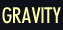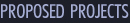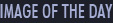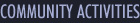The terms gravity and gravitation are mostly interchangeable in everyday use, but a distinction is made in scientific circles. "Gravitation" is a general term describing the phenomenon by which bodies with mass are attracted to one another, while "gravity" refers specifically to the net force exerted by the Earth on objects in its vicinity as well as by other factors, such as the Earth's rotation.

In everyday life, gravitation is most commonly thought of as the agency which lends weight to objects with mass. Gravitation compels dispersed matter to come together, thus accounting for the existence of the Earth, the Sun, and most of the macroscopic objects in the universe. It is the force for keeping the Earth and the other planets in their orbits around the Sun and for keeping the Moon in its orbit around the Earth. Modern physics describes gravitation using the general theory of relativity, in which gravitation is a consequence of the curvature of space time which governs the motion of objects.

### Earth’s Gravity

Every object with mass is surrounded by its own gravitational field, which exerts an attractive force on all objects. Assuming a spherically symmetrical planet, the strength of this field at any given point is proportional to the planetary body's mass and inversely proportional to the square of the distance from the center of the body.

The strength of the gravitational field is numerically equal to the acceleration of objects under its influence, and its value at the Earth's surface, denoted g, is approximately expressed below as the standard average.

g = 9.81 m/s2 = 32.2 ft/s2

This means that, ignoring air resistance, an object falling freely near the Earth's surface increases its velocity with 9.81 m/s for each second of its descent. Thus, an object starting from rest will attain a velocity of 9.81 m/s after one second, 19.6 m/s after two seconds, and so on, adding 9.81 m/s to each resulting velocity. Also, again ignoring air resistance, any and all objects, when dropped from the same height, will hit the ground at the same time.

According to Newton's 3rd Law, the Earth itself experiences an equal and opposite force to that acting on the falling object, meaning that the Earth also accelerates towards the object (until the object hits the earth, then the Law of Conservation of Energy states that it will move back with the same acceleration with which it initially moved forward, canceling out the two forces of gravity.). However, because the mass of the Earth is huge, the acceleration of the Earth by this same force is negligible, when measured relative to the system's center of mass.

### Anti-gravity

In physical cosmology, astronomy and celestial mechanics, anti-gravity is the idea of creating a place or object that is free from the force of gravity. It does not refer to the lack of weight experienced in free fall or orbit, or to balancing the force of gravity with some other force, such as electromagnetism. Instead, anti-gravity requires that the fundamental causes of the force of gravity be made either not present or not applicable to the place or object through some kind of technological intervention. Anti-gravity is a recurring concept in science fiction, particularly in the context of spacecraft propulsion. The concept was first introduced formally in H.G. Wells' The First Men in the Moon, and has been a favorite item of imaginary technology since that day.

In the first mathematically accurate description of gravity, Newton's law of universal gravitation, gravity was an external force transmitted by unknown means. However in the early part of the 20th century Newton's model was replaced by the more general and complete description known as general relativity. In general relativity, gravity is not a force in the traditional sense of the word, but the result of the geometry of space itself. These geometrical solutions always cause attractive "forces". The term "anti-gravity" is also sometimes used to refer to hypothetical reaction less propulsion drives based on certain solutions to general relativity, although these do not oppose gravity as such.

There are numerous newer theories that add onto general relativity or replace it outright, and some of these appear to allow anti-gravity-like solutions.

Search:Find out what Australian scientists have planned as our future aerospace activities.
MoreView Image GalleryThe Australian Space Agency has numerous amateur activities and associations that are open for community involvement. Join other enthusiasts and share the excitement of Space.
More